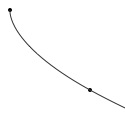### Reference | findpath()

Syntax
`findpath(list, curvature =1.0)`

DescriptionConstructs a fluid path from a list of coordinates. Each element in the list is a 2-tuple defining the x-coordinate and the y-coordinate. If the curve has more than three points, the curvature parameter offers some control on how separate segments are stitched together: from straight lines (0.0) to smooth curves (1.0).
Returns BezierPath containing the constructed path
Tutorial Paths

Example```points = [(10, 10), (90, 90), (350, 200)] for x, y in points: oval(x-2, y-2, 4, 4)   nofill() stroke(0.2) autoclosepath(False) path = findpath(points) drawpath(path)```